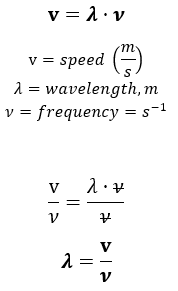# Problem: The speed of sound in air is 344 m/s at room temperature. The lowest frequency of a large organ pipe is 30 s-1 and the highest frequency of a piccolo is 1.5 x 104 s- 1. Find the difference in wavelength between these two sounds.

###### FREE Expert Solution

Calculate wavelength using the following equation:Calculate the wavelength of a large organ pipe:

81% (395 ratings)###### Problem Details

The speed of sound in air is 344 m/s at room temperature. The lowest frequency of a large organ pipe is 30 s-1 and the highest frequency of a piccolo is 1.5 x 104 s- 1. Find the difference in wavelength between these two sounds.